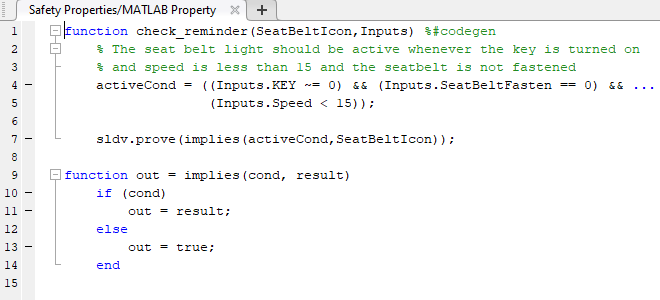sldv.assume

Proof assumption function for Stateflow charts and MATLAB Function blocks

Description

example

sldv.assume(expr) specifies that expr is true for every evaluation while proving properties. Use any valid Boolean expression for expr.

This function has no output and no impact on its parenting function, other than any indirect side effects of evaluating expr. If you issue this function from the MATLAB® command line, the function has no effect.

Intersperse sldv.assume proof assumptions within MATLAB code or separate the assumptions into a verification script.

The Proof assumptions option in the Property proving pane applies to the proof assumptions represented by the sldv.assume function and by the Proof Assumption block.

Examples

collapse all

Specify a property proof objective and proof assumption in the sldvdemo_sbr_verification model by using a MATLAB Function block.

Open the sldvdemo_sbr_verification model and save it as ex_sldvdemo_sbr_verification.

Open the Safety Properties subsystem.Open the MATLAB Property block, which is a MATLAB Function block.At the end of the check_reminder function definition, add sldv.assume(Inputs.KEY==0 | 1); so that the last two lines of the function definition are:

sldv.prove(implies(activeCond, SeatBeltIcon));
sldv.assume(Inputs.KEY==0 | 1);

To save the updated code, in the Editor tab, click Save and close the editor.

To prove the safety properties, in the Simulink® Editor, select the Safety Properties subsystem. On the Design Verifier tab, click .

Alternatively, in the Simulink Editor, you can right-click the Safety Properties subsystem and select Design Verifier > Prove Subsystem Properties.

Input Arguments

collapse all

MATLAB expression, for example, x > 0.

Alternatives

Instead of using the sldv.assume function, you can insert a Proof Assumption block in your model. Using sldv.assume instead of a Proof Assumption block offers several benefits, described in What Is Property Proving?.

When proving models by using MATLAB for code generation, you can also constrain signal values without using the sldv.assume function. Using sldv.assume instead of directly using MATLAB for code generation eliminates the need to:

• Express the assumption by using a Simulink block.

• Explicitly connect the assumption output to a Simulink block.